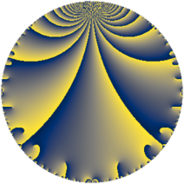# Properties

 Label 23.3.bLevel $23$ Weight $3$ Character orbit 23.b Rep. character $\chi_{23}(22,\cdot)$ Character field $\Q$ Dimension $3$ Newform subspaces $1$ Sturm bound $6$ Trace bound $0$

# Related objects

## Defining parameters

 Level: $$N$$ $$=$$ $$23$$ Weight: $$k$$ $$=$$ $$3$$ Character orbit: $$[\chi]$$ $$=$$ 23.b (of order $$2$$ and degree $$1$$) Character conductor: $$\operatorname{cond}(\chi)$$ $$=$$ $$23$$ Character field: $$\Q$$ Newform subspaces: $$1$$ Sturm bound: $$6$$ Trace bound: $$0$$

## Dimensions

The following table gives the dimensions of various subspaces of $$M_{3}(23, [\chi])$$.

Total New Old
Modular forms 5 5 0
Cusp forms 3 3 0
Eisenstein series 2 2 0

## Trace form

 $$3 q + 12 q^{4} - 33 q^{6} - 21 q^{8} + 27 q^{9} + O(q^{10})$$ $$3 q + 12 q^{4} - 33 q^{6} - 21 q^{8} + 27 q^{9} + 3 q^{12} + 48 q^{16} + 39 q^{18} - 69 q^{23} - 132 q^{24} + 75 q^{25} + 87 q^{26} - 114 q^{27} - 84 q^{32} + 255 q^{36} - 42 q^{39} + 231 q^{48} + 147 q^{49} - 309 q^{52} - 297 q^{54} - 273 q^{58} + 78 q^{59} + 303 q^{62} - 45 q^{64} - 33 q^{72} + 399 q^{78} + 243 q^{81} - 129 q^{82} + 246 q^{87} - 276 q^{92} - 546 q^{93} - 57 q^{94} - 21 q^{96} + O(q^{100})$$

## Decomposition of $$S_{3}^{\mathrm{new}}(23, [\chi])$$ into newform subspaces

Label Dim $A$ Field CM Traces $q$-expansion
$a_{2}$ $a_{3}$ $a_{5}$ $a_{7}$
23.3.b.a $3$ $0.627$ 3.3.621.1 $$\Q(\sqrt{-23})$$ $$0$$ $$0$$ $$0$$ $$0$$ $$q+(\beta _{1}+\beta _{2})q^{2}+(-2\beta _{1}-\beta _{2})q^{3}+(4+\cdots)q^{4}+\cdots$$